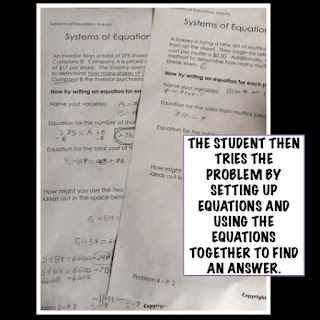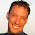Do you ever give those "zoo" problems?  I don't really know what they are called but they are the ones where the zookeeper has some flamingos and some elephants. There are 15 animals in total and 38 legs. How many of each animal are there? (Or if you are feeling other worldly maybe you use cyclops and fairies and you ask how many eyes there are.)

Anyway I love these problems when we are working on problem solving strategies because there are many ways to approach - make a table, guess and check, solve a simpler problem, and draw it are just a few that come to mind.  But in my more advanced math, students learn to go beyond these strategies and use more formal algebraic approaches.

Yet, I like to start with problem solving approaches particularly when teaching systems of equations. Although students know what equations are, this whole systems idea can seem kind of abstract, particularly when working primarily with variables.

So when a student gets to systems of equations, they start with an inquiry that is familiar - a problem solving question that just happens to also be a systems of equations problem.• A student solves an inquiry problem first using any method they choose.
• Next, the student sets up equations and problem solve how they might use the equations together to find a solution.
• At the next lesson when the student is exploring formal strategies for systems of equations such as substitution and elimination, the strategies are a little more logical because students really have already used these strategies.

And although this post was specifically related to inquiry with systems of equations the basic teaching strategies can be used across the curriculum:

• Create an opportunity for inquiry
• Give students the chance to problem solve (and this is not about getting the answer now)
• Provide formal strategies related to the inquiry activity.
What kind of inquiry or approach do you use when teaching new math concepts?  Join the discussion in the comments below and checkout some inquiry resources for your classroom.

### Share:

1.2.3.